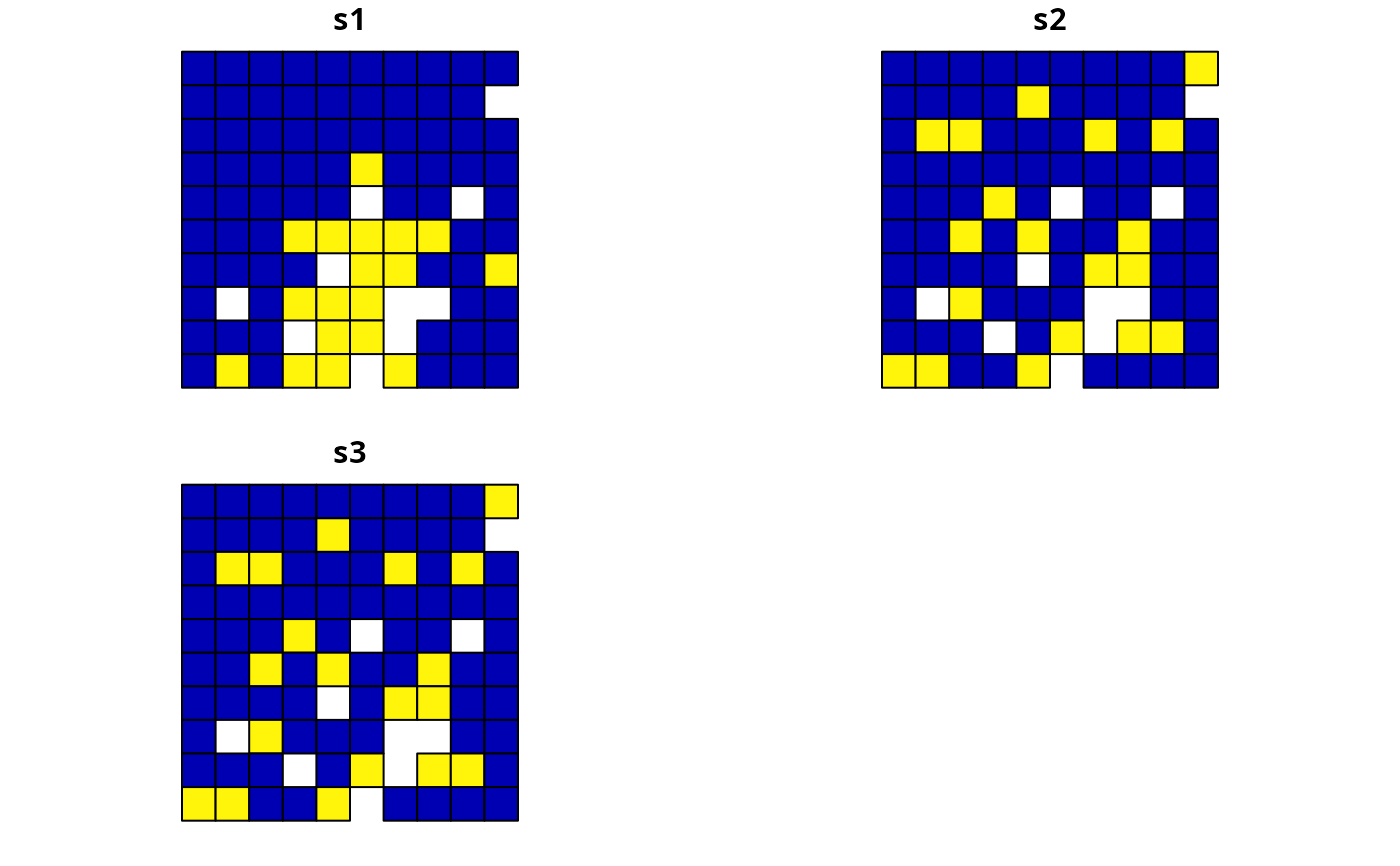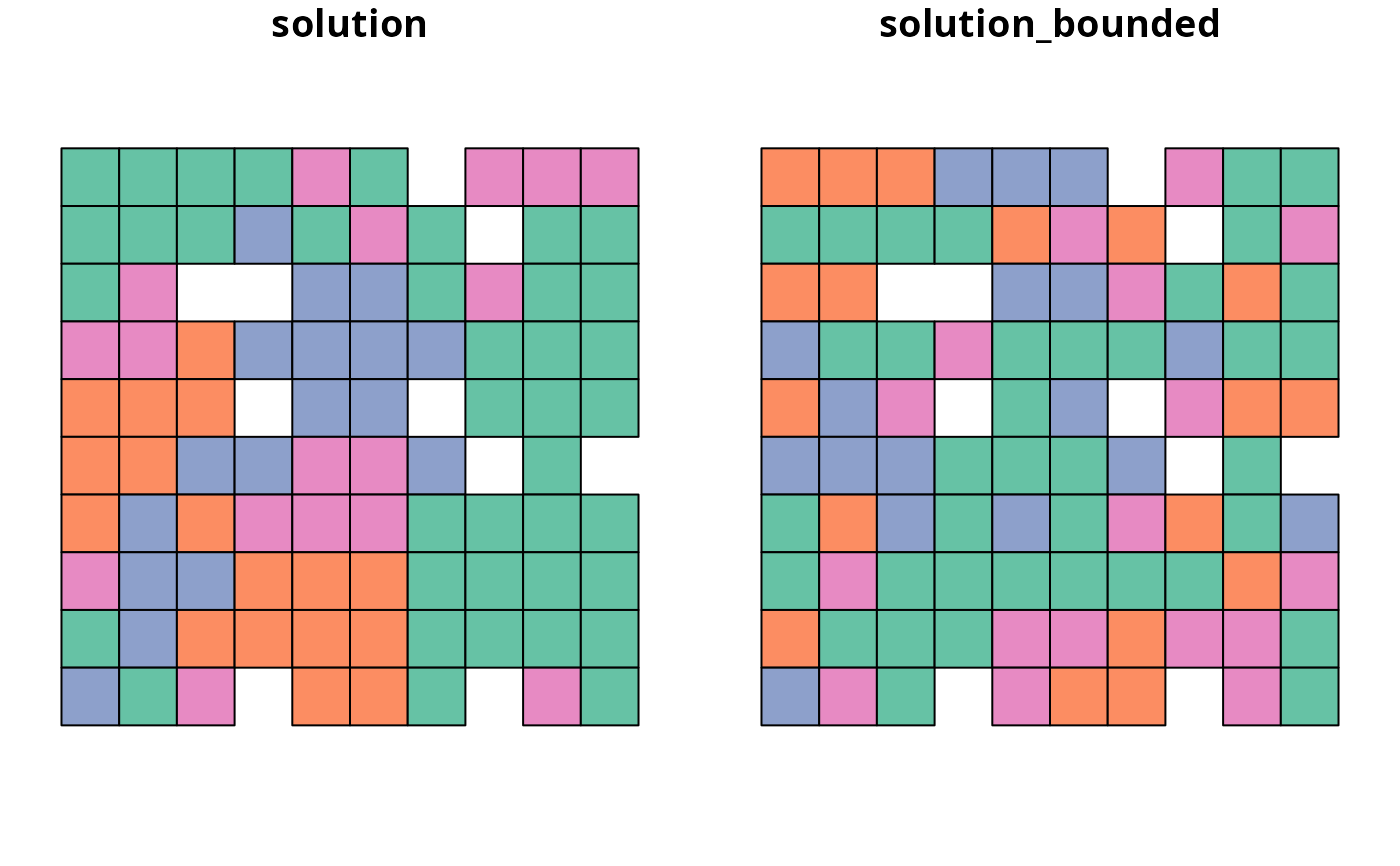Add constraints to a conservation planning problem to ensure that the planning unit values (e.g., proportion, binary) in a solution range between specific lower and upper bounds. This function offers more fine-grained control than the add_manual_locked_constraints() function and is is most useful for problems involving proportion-type or semi-continuous decisions.

## Usage

add_manual_bounded_constraints(x, data)

# S4 method for ConservationProblem,data.frame

# S4 method for ConservationProblem,tbl_df
add_manual_bounded_constraints(x, data)

## Arguments

x

problem() object.

data

data.frame or tibble::tibble() object. See the Data format section for more information.

## Value

An updated problem() object with the constraints added to it.

## Data format

The argument to data should be a data.frame with the following columns:

pu

integer planning unit identifier.

zone

character names of zones. Note that this argument is optional for arguments to x that contain a single zone.

lower

numeric values indicating the minimum value that each planning unit can be allocated to in each zone in the solution.

upper

numeric values indicating the maximum value that each planning unit can be allocated to in each zone in the solution.

See constraints for an overview of all functions for adding constraints.

Other constraints: add_contiguity_constraints(), add_feature_contiguity_constraints(), add_linear_constraints(), add_locked_in_constraints(), add_locked_out_constraints(), add_mandatory_allocation_constraints(), add_manual_locked_constraints(), add_neighbor_constraints()

## Examples

# \dontrun{
# set seed for reproducibility
set.seed(500)

sim_pu_polygons <- get_sim_pu_polygons()
sim_features <- get_sim_features()
sim_zones_pu_polygons <- get_sim_zones_pu_polygons()
sim_zones_features <- get_sim_zones_features()

# create minimal problem
p1 <-
problem(sim_pu_polygons, sim_features, "cost") %>%

# create problem with locked in constraints using add_locked_constraints

# create identical problem using add_manual_bounded_constraints
bounds_data <- data.frame(
pu = which(sim_pu_polygons$locked_in), lower = 1, upper = 1 ) p3 <- p1 %>% add_manual_bounded_constraints(bounds_data) # solve problems s1 <- solve(p1) s2 <- solve(p2) s3 <- solve(p3) # create object with all solutions s4 <- sf::st_sf( tibble::tibble( s1 = s1$solution_1,
s2 = s2$solution_1, s3 = s3$solution_1
),
geometry = sf::st_geometry(s1)
)

# plot solutions
## s1 = none locked in
## s2 = locked in constraints
## s3 = manual bounds constraints
plot(s4)# create minimal problem with multiple zones
p5 <-
problem(
sim_zones_pu_polygons, sim_zones_features,
c("cost_1", "cost_2", "cost_3")
) %>%
add_relative_targets(matrix(runif(15, 0.1, 0.2), nrow = 5, ncol = 3)) %>%

# create data.frame with the following constraints:
# planning units 1, 2, and 3 must be allocated to zone 1 in the solution
# planning units 4, and 5 must be allocated to zone 2 in the solution
# planning units 8 and 9 must not be allocated to zone 3 in the solution
bounds_data2 <- data.frame(
pu = c(1, 2, 3, 4, 5, 8, 9),
zone = c(rep("zone_1", 3), rep("zone_2", 2), rep("zone_3", 2)),
lower = c(rep(1, 5), rep(0, 2)),
upper = c(rep(1, 5), rep(0, 2))
)

# print bounds data
print(bounds_data2)
#>   pu   zone lower upper
#> 1  1 zone_1     1     1
#> 2  2 zone_1     1     1
#> 3  3 zone_1     1     1
#> 4  4 zone_2     1     1
#> 5  5 zone_2     1     1
#> 6  8 zone_3     0     0
#> 7  9 zone_3     0     0

# create problem with added constraints

# solve problem
s5 <- solve(p5)
s6 <- solve(p6)

# create two new columns representing the zone id that each planning unit
# was allocated to in the two solutions
s5$solution <- category_vector(sf::st_drop_geometry( s5[, c("solution_1_zone_1","solution_1_zone_2", "solution_1_zone_3")] )) s5$solution <- factor(s5$solution) s5$solution_bounded <- category_vector(sf::st_drop_geometry(
s6[, c("solution_1_zone_1", "solution_1_zone_2", "solution_1_zone_3")]
))
s5$solution_bounded <- factor(s5$solution_bounded)

# plot solutions
plot(s5[, c("solution", "solution_bounded")], axes = FALSE)# }